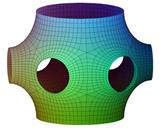Springe direkt zu Inhalt

# Matheon 2002-2006: F1 - Discrete Differential Geometry

Institution:

AG Mathematical Geometry Processing
FB Mathematik und Informatik

Principal Investigator:
Funding:
DFG Research Center Matheon "Mathematics for key technologies"
Term:
Jun 01, 2002 — May 31, 2006Matheon 2002-2006: F1 - Discrete Differential Geometry

This is another project within the DFG Research Center MATHEON - "Mathematics for key technologies".  The main goal of this project is the discretization of classical differential geometry: to find proper discrete analogs of differential geometric objects, notions, and methods, and to develop a corresponding theory. Such a discrete differential geometry has applications in computer graphics, numerics, and visualization.

The main goal of this project is the discretization of classical differential geometry: to find proper discrete analogs of differential geometric objects, notions, and methods, and to develop a corresponding theory. Such a discrete differential geometry has applications in computer graphics, numerics, and visualization.